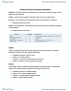STAT151 Study Guide - Final Guide: Nypd Blue, Fair Coin, Box Plot

363 views53 pages
School
Department
Coursesalmonmarten267 and 7 others unlocked4
Verified Note
4 documents

Document Summary

Chapter 23: comparing counts and tests and confidence intervals. This chapter covers inferential statistics, its objective is to use sample data to obtain results about the whole population. In a first step, the goal is to describe an underlying population. Since the populations are described in form of models that are characterized by parameters (mean and standard deviation or probability p for the event of interest). At this time we will estimate those characteristics. There are two different approaches for estimating: point estimation and. For point estimation, you give one value for a characteristic, which is hopefully close to the true unknown value. For interval estimation, you give an interval of likely values, where the width of the interval will depend on the confidence you require to have in this interval. Since we base our statement just on a sample, we see later how to give a measure of accuracy or confidence for the estimate.

Get access

\$8 USD/m\$10 USD/m
Billed \$96 USD annuallyHomework Help
Study Guides
Textbook Solutions
Class Notes
Textbook Notes
Booster Class